Open In App

# Subtracting Fractions

Subtracting fractions with unlike denominators can be a daunting task for many students when it is introduced to them, but it doesn’t have to be. With a few simple steps and some practice, you can become proficient in subtracting fractions with ease. In this article, we will guide you through the process of subtracting fractions with unlike denominators and provide you with some solved examples to help you master this important math skill. Additionally, we will cover some common mistakes to avoid and provide you with practice problems to reinforce your learning.

## Fractions Definition

Fractions are a fundamental concept in mathematics that helps us represent a part of a whole. In other words, if we divide an object into b equal parts and take its a (<b) part, then this can easily be represented by the concept of fraction i.e., a/b. Formally fractions are defined as

A fraction is a mathematical expression that represents a quantity that is not a whole number. It is typically written as a/b.

Where,

• a is the numerator, and
• b ≠ 0 is the denominator.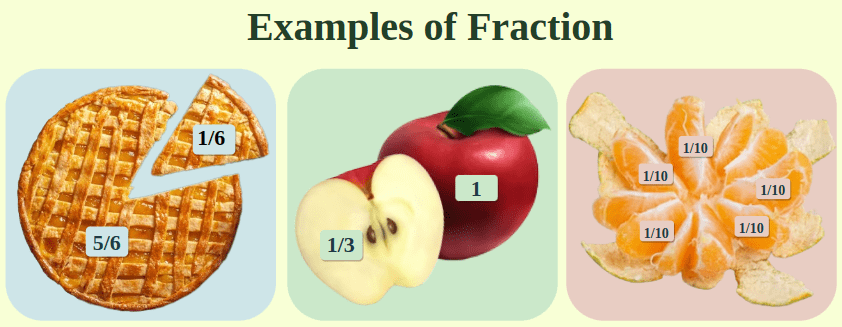### What are Numerators and Denominators in Fractions?

In fractions, there are two parts i.e., Numerator and Denominator. The denominator represents the total number of equal parts which need to complete one whole object whereas the numerator represents the number of parts taken into consideration for fraction. For example, in a fraction of 3/4, the denominator is 4, which means the whole is divided into four equal parts, and we are considering three of them thus, the numerator is 3.

### What are Unlike Denominators?

Denominators can be classified as like and unlike denominators. When the denominator for two or more fractions is the same then those fractions are called fractions with like denominators and when denominators for two or more fractions are not the same then the fractions are known as fractions with unlike denominators.

For example, if we consider fractions 1/4, 3/4, and 5/4, these all have the same denominator i.e., 4. Thus these are examples of like denominators and if we consider fractions like 3/4, 2/5, and 7/11, these all have different denominators i.e., 4, 5, and 11. Thus, these fractions are examples of, unlike denominators in fractions.

## Subtracting Fractions with Unlike Denominators: Step-by-Step Method

When subtracting fractions with different denominators we need to use the following steps:

Step 1: Identify the unlike denominators of the fractions you want to subtract.

Step 2: Find the least common multiple (LCM) of the denominators i.e., the smallest number that is divisible by all denominators.

Step 3: Multiply the numerator and denominator by the factor of LCM to make denominators equal.

Step 4: Subtract the numerators of the fractions, keeping the LCM as the denominator.

Step 5: Simplify the result, if possible.

### Example of Subtracting Fractions with Unlike Denominators

Let’s consider some solved examples of subtraction of fractions with, unlike denominators to understand the concept and method better.

Example: Subtract 3/5 from 1/3.

Solution:

Step 1: Identify the unlike denominators of the fractions you want to subtract.

The denominators are 5 and 3, which are unlike.

Step 2: Find the least common multiple (LCM) of the denominators.

Multiples of 5: 5, 10, 15, 20, 25, 30, 35, 40, 45, 50, 55, 60, …

Multiples of 3: 3, 6, 9, 12, 15, 18, 21, 24, 27, 30, 33, 36, 39, 42, 45, 48, 51, 54, 57, 60, …

The LCM of 5 and 3 is 15.

Step 3: Multiply the numerator and denominator by the factor of LCM to make denominators equal.

• 1/3 = (1 × 5)/(3 × 5) = 5/15
• 3/5 = (3 × 3)/(5 × 3) = 9/15

Step 4: Subtract the numerators of the fractions, keeping the LCM as the denominator.

9/15 – 5/15 = 4/15

Step 5: Simplify the result, if possible.

4/15 cannot be simplified any further, so the final answer is 4/15.

Let’s consider one more example for better understanding.

Example: Consider two numbers 1/3 and 4/5. Subtract a smaller fraction from the larger one.

Solution:

Given two fractions: 1/3 and 4/5.

To perform Subtraction or even to compare the two numbers, we will need to have a common denominator for both of them.

Given First Fractional Number: 1/3

Given Second Fractional Number: 4/5

Numbers in Denominator: 3 for the first number and 5 for the second number.

We will find LCM of the numbers 3 and 5

The LCM of 3 and 5 is 15.

So, to attain 15 as the denominator, the multiplying factor for numerator and denominator for the first fractional number will be 5. Similarly, the multiplying factor for numerator and denominator for the second fractional number will be 3

First Fractional Number: (1×5)/(3×5) = 5/15

Second Fractional Number: (4×3)/(5×3) = 12/15

Now since the denominator is same, we will compare the numerators. Clearly, 12/15 is greater than 5/15. So, we will subtract 5/15 from 12/15.

Second Fractional Number > First Fractional Number

Second Fractional Number – First Fractional Number

(12/15) – (5/15) = 7/15

## Understanding Subtracting Fractions with Unlike Denominators

Let’s consider an example of 2/3 – 1/4 for this illustration.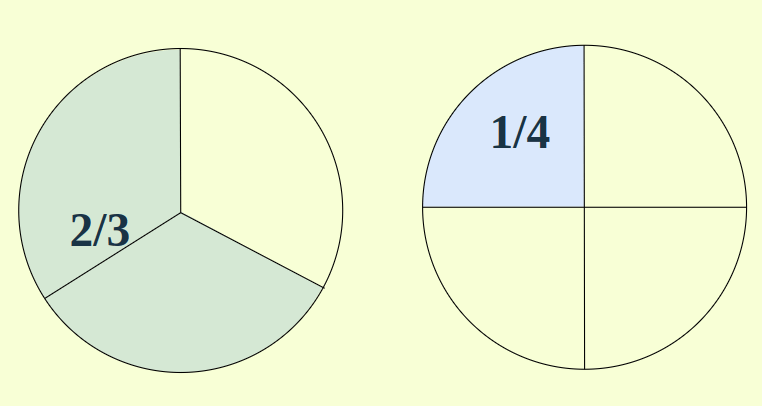To subtract the 1/4 from 2/3, we take the LCM of the denominators which can be represented by partitioning the circle into equal parts as follows: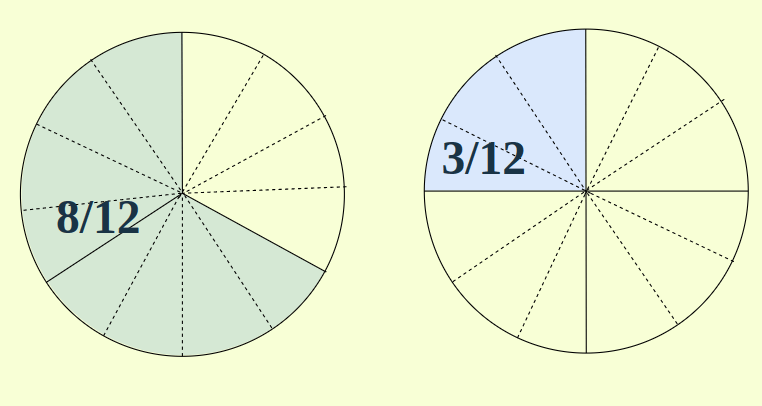Now, each partition of both circles represents an equal area, thus we can subtract them as a single unit.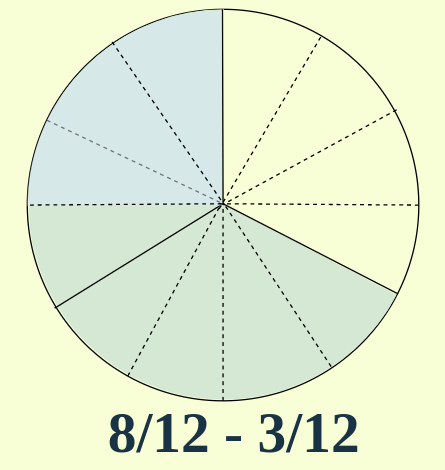Therefore, 8/12 – 3/12 = 5/12 i.e., 5 parts out of 12 equal parts.

## Subtracting Fractions with Like Denominators

Subtracting fractions with like denominators is comparatively easy as in this you don’t need to equalize the denominator by taking LCM and multiplying the required factor in the denominator. One should follow the below-mentioned steps for subtracting fractions with like denominators.

Step 1: First identify larger and smaller fractions. If two fractions have the same denominators then the fraction with a larger denominator is larger.

Step 2: Arrange the fraction for subtraction and take the LCM of the denominator. The LCM of the same number is the number itself.

Step 3: Since, the LCM is same the factor on dividing LCM and the denominator is 1 with which multiply the numerators.

Step 4: Subtract the numerators mentioned in the fraction and write the same denominator to get the answer.

Example: Find the difference between 5/4 and 3/4

Solution:

Step 1: Since the denominator is the same therefore fraction with a larger denominator is larger. Hence, 5/4 > 3/4.

Step 2: Arrange the fraction 5/4 – 3/4. Now the LCM of 4 will be 4.

Step 3: On dividing LCM 4 by denominator 4 we get 1 which we will multiply with numerators 5 and 3.

Step 4: Hence, on multiplying with 1 we get (5-3)/4 as the fraction. Thus 2/4 is our desired result.

Note: If the fraction can be reduced to simpler forms, reduce it. For example, here we can reduce 2/4 to 1/2.

## Understanding Subtracting Fractions With Like Denominators

In this section, we will understand subtracting fractions having the same denominators with the help of the pictures. For Example, let us assume we have to subtract 3/4 and 1/4

The image added below shows 3/4 of a whole and 1/4 of a whole.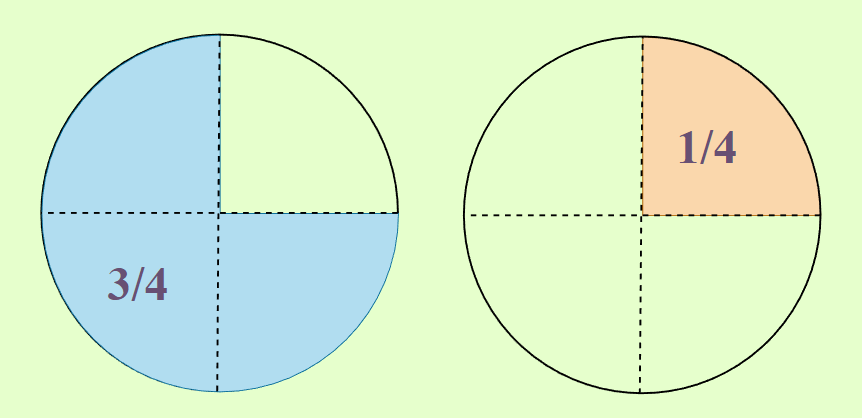Since the denominator is the same for both we don’t need to worry about LCM as LCM will be the same as the denominator, hence no change in the denominator is required. Hence, the image attached will not be divided into more parts as we did in the case of, unlike denominators.

Now the only thing we have to do is to subtract the numerators while keeping the denominators the same to get the desired result. Also, check if the obtained result can be further reduced to simpler forms to get the final result. Hence, our result will be (3 – 1)/4 = 2/4 = 1/2 which is shown in the figure below.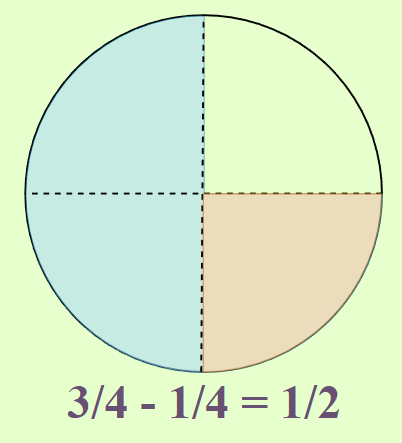## Some Common Mistakes While Subtracting Fractions

Some Common mistakes while subtracting fractions are as follows:

• Error in finding common denominator(LCM of denominators): Calculating LCM for two or more numbers seems easy but sometimes you can find errors in the calculation when there are more than two numbers. So, calculate LCM carefully. Also, calculate LCM very first as either you need to add or subtract fractions with unlike denominators requires the denominators to be the same.
• Simplifying too soon: When subtracting fractions, it is important to simplify the resulting fraction only after you have subtracted the numerators and found a common denominator. Simplifying too soon can lead to inconvenience and incorrect answers.

## Sample Problems on Subtract Fractions

Problem 1: Subtract 1/3 from 1/2.

Solution:

Numbers in Denominator: 3 for the first number and 2 for the second number.

We will find LCM of the numbers 3 & 2

The LCM of 3 & 2 is 6.

So, to attain 6 as the denominator, the multiplying factor for the numerator and denominator for the first fractional number will be 2. Similarly, the multiplying factor for the numerator and denominator for the second fractional number will be 3

First Fractional Number: (1*2)/(3*2)

= 2/6

Second Fractional Number: (1*3)/(2*3)

= 3/6

So, (1*3)/(2*3) – (1*2/3*2)

= (3/6) – (2/6)

= 1/6

Problem 2: Subtract 1/4 from 1/3.

Solution:

Numbers in Denominator: 4 for the first number and 3 for the second number.

We will find LCM of the numbers 4 & 3

The LCM of 4 & 3 is 12.

So, to attain 12 as the denominator, the multiplying factor for the numerator and the denominator for the first fractional number will be 3. Similarly, the multiplying factor for the numerator and denominator for the second fractional number will be 4

First Fractional Number: (1*3)/(4*3)

= 3/12

Second Fractional Number: (1*4)/(3*4)

= 4/12

So, [(1*4)/(3*4) – (1*3)/(4*3)]

= (4/12) – (3/12)

= 1/12

Problem 3:Subtract 1/4 from 1/2.

Solution:

Numbers in Denominator: 4 for the first number and 2 for the second number.

We will find LCM of the numbers 4 & 2

The LCM of 4 & 2 is 4.

So, to attain 4 as the denominator, the multiplying factor for the numerator and the denominator for the first fractional number will be 1. Similarly, the multiplying factor for the numerator and denominator for the second fractional number will be 2

First Fractional Number: (1*1)/(4*1)

= 1/4

Second Fractional Number: (1*2)/(2*2)

= 2/4

So, [(1*2)/(2*2) – (1*1)/(4*1)]

= (2/4) – (1/4)

= 1/4

Problem 4: Subtract 1/5 from 1/2.

Solution:

Numbers in Denominator: 5 for the first number and 2 for the second number.

We will find LCM of the numbers 5 & 2

The LCM of 5 & 2 is 10.

So, to attain 10 as the denominator, the multiplying factor for the numerator and the denominator for the first fractional number will be 2. Similarly, the multiplying factor for the numerator and denominator for the second fractional number will be 5

First Fractional Number: (1*2)/(5*2)

= 2/10

Second Fractional Number: (1*5)/(2*5)

= 5/10

So,

= (5/10) – (2/10)

= 3/10

Problem 5: Subtract 1/5 from 1/4.

Solution:

Numbers in Denominator: 5 for the first number and 4 for the second number.

We will find LCM of the numbers 5 & 4

The LCM of 5 & 4 is 20.

So, to attain 20 as the denominator, the multiplying factor for the numerator and denominator for the first fractional number will be 4. Similarly, the multiplying factor for the numerator and denominator for the second fractional number will be 5

First Fractional Number: (1*4)/(5*4)

= 4/20

Second Fractional Number: (1*5)/(4*5)

= 5/20

So,

= (5/20) – (4/20)

= 1/20

## FAQs on Subtract Fractions with Unlike Denominators

### Q1: What are Fractions with, Unlike Denominators?

Fractions with unlike denominators are fractions that have different numbers in their denominators. For example, 1/3 and 1/4 are fractions with unlike denominators.

### Q2: What is Subtracting Fractions with, Unlike Denominators?

Subtracting fractions with unlike denominators is the process of finding the difference between two fractions that have different numbers in their denominators.

### Q3: How do Subtract Fractions with Different Denominators?

To subtract fractions with unlike denominators, you need to find a common denominator for the fractions, then convert each fraction to an equivalent fraction with the common denominator. Once the fractions have the same denominator, you can subtract the numerators and simplify the result if possible.